# Introduction to Fitting PHA Spectra## Overview

#### Synopsis:

The basic steps used in fitting spectral data are illustrated in this thread. The data used herein were created by running the Creating ACIS Spectra for Pointlike Sources thread.

There are many options and variables that may affect how this process is applied to your data; for a more detailed explanation of the steps, see the following threads:

For a detailed explanation of the fitting concepts behind X-ray spectral analysis in Sherpa, see the document Spectral Fitting on the Sherpa References page.

Before fitting ACIS data sets with restricted pulse-height ranges, please read the CIAO caveat page "Spectral analyses of ACIS data with a limited pulse-height range."

Last Update: 28 Nov 2022 - updated screen output for CIAO 4.15.

## Load the Spectrum & Instrument Responses

sherpa> load_pha("3c273.pi")
statistical errors were found in file '3c273.pi'
but not used; to use them, re-read with use_errors=True
statistical errors were found in file '3c273_bg.pi'
but not used; to use them, re-read with use_errors=True


Since the RESPFILE, ANCRFILE, and BACKFILE header keywords were updated in the spectrum file, the response files (RMF and ARF) and background file are automatically read in as well. If the default dataset ID of "1" is to be used, it does not need to be explicitly included in the load function; only the data filenames are required in this case.

Sherpa issued a warning about systematic and statistical errors, which were not loaded. The statistical errors are calculated using the appropriate fit statistics set with set_stat in the Sherpa session. The standard treatment of systematic errors supplied with load_syserror is to add the array of systematic errors in quadrature to the statistical errors. Advanced methods to account for non-linear calibration uncertainties described in Lee et al. (2011) are available within pyblocxs Bayesian functions. However, they require calibration products that are not available at this moment.

If Sherpa does not automatically read in the background and response files, read them manually:

sherpa> load_arf("3c273.arf")


Use show_all, show_data, and show_bkg to get the status of the Sherpa session. Some additional commands are used to get the total number of counts and counts rates calculated from the data.

sherpa> show_all()
Data Set: 1
Filter: 0.0015-14.9504 Energy (keV)
Bkg Scale: 0.134921
Noticed Channels: 1-1024
name           = 3c273.pi
channel        = Float64
counts         = Float64
staterror      = None
syserror       = None
bin_lo         = None
bin_hi         = None
grouping       = Int16
quality        = Int16
exposure       = 38564.608926889
backscal       = 2.5264364698914e-06
areascal       = 1.0
grouped        = True
subtracted     = False
units          = energy
rate           = True
plot_fac       = 0
response_ids   = 
background_ids = 

RMF Data Set: 1:1
name     = 3c273.rmf
energ_lo = Float64
energ_hi = Float64
n_grp    = UInt64
f_chan   = UInt32
n_chan   = UInt32
matrix   = Float64
e_min    = Float64
e_max    = Float64
detchans = 1024
offset   = 1
ethresh  = 1e-10

ARF Data Set: 1:1
name     = 3c273.arf
energ_lo = Float64
energ_hi = Float64
specresp = Float64
bin_lo   = None
bin_hi   = None
exposure = 38564.141454905
ethresh  = 1e-10

Background Data Set: 1:1
Filter: 0.0015-14.9504 Energy (keV)
Noticed Channels: 1-1024
name           = 3c273_bg.pi
channel        = Float64
counts         = Float64
staterror      = None
syserror       = None
bin_lo         = None
bin_hi         = None
grouping       = Int16
quality        = Int16
exposure       = 38564.608926889
backscal       = 1.872535141462e-05
areascal       = 1.0
grouped        = True
subtracted     = False
units          = energy
rate           = True
plot_fac       = 0
response_ids   = 
background_ids = []

Background RMF Data Set: 1:1
name     = 3c273.rmf
energ_lo = Float64
energ_hi = Float64
n_grp    = UInt64
f_chan   = UInt32
n_chan   = UInt32
matrix   = Float64
e_min    = Float64
e_max    = Float64
detchans = 1024
offset   = 1
ethresh  = 1e-10

Background ARF Data Set: 1:1
name     = 3c273.arf
energ_lo = Float64
energ_hi = Float64
specresp = Float64
bin_lo   = None
bin_hi   = None
exposure = 38564.141454905
ethresh  = 1e-10

sherpa> data_sum = calc_data_sum(id=1)  # total counts (or values) in the data
sherpa> print(data_sum)
736.0

sherpa> data_cnt_rate = calc_data_sum()/get_exposure(id=1)  # calculating count rate in cts/sec
sherpa> print(data_cnt_rate)
0.019084855790844735

sherpa> bkg_sum = calc_data_sum(bkg_id=1)   # total counts (or values) in the background data
sherpa> print(bkg_sum)
216.0

sherpa> bkg_cnt_rate = calc_data_sum(bkg_id=1)/get_exposure(bkg_id=1)  # calculating background count rate in cts/sec

sherpa> print(bkg_cnt_rate)
0.005600990286443563


Plot the data:

sherpa> plot_data()


The data are plotted in energy space—as seen in Figure 1—since the instrument model provides the information necessary to compute the predicted counts for each bin. In general, the units of the x-axis are determined by the value in the units field of the data, which may be accessed with 'print(get_data().units)' or show_filter, and modified with set_analysis.

### Figure 1: Plot of source spectrum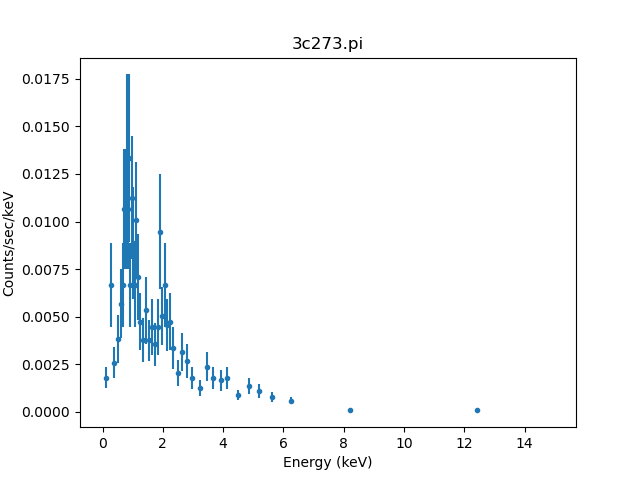## Filter the Data & Subtract the Background

The CIAO 'Why' topic on Choosing an Energy Filter contains information on selecting energy range for spectral modeling. We can use the Sherpa ignore or notice functions to select the energy range between 0.3 and 6.0 keV. These functions are applied to all data sets. The other two functions, notice_id()/ignore_id(), require explicit input of the source data set ID, as the first argument; the second argument defines the lower energy of the range, and the third the higher energy of the range. These are useful for multiple data sets requiring different filters. The data between 0.3 and 6.0 keV will be noticed with the command:

sherpa> notice(0.3, 6.0)
dataset 1: 0.00146:14.9504 -> 0.2482:6.57 Energy (keV)Note

The notice_id filter will automatically be applied to the associated background data when the background data set ID (bkg_id) parameter is not used, as in the example in this thread. A different filter for the background may be set by issuing the notice_id or ignore_id command with the bkg_id entered as the fourth argument to the function.

with the change in the filter also reported, as bounded by the appropriate bin edges from the response energy grid. At this point, we also opt to subtract the background data:

sherpa> subtract()

sherpa> plot_data()


Figure 2 shows the resulting plot.

### Figure 2: Source spectrum, filtered and background-subtracted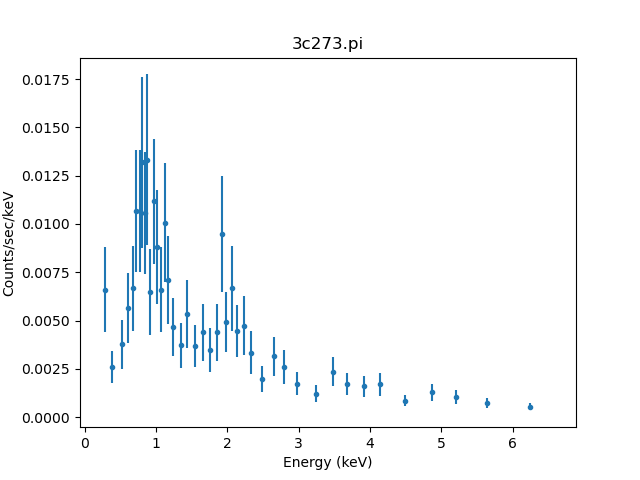### Figure 2: Source spectrum, filtered and background-subtracted

The axis scaling for all plots created in the current Sherpa session may be changed to log by calling set_xlog and set_ylog with no arguments (and changed back to linear with set_xlinear/set_ylinear):

sherpa> set_xlog()
sherpa> set_ylog()Tip

To set the plot axis scaling for a specific type of plot, e.g., model, data, or fit plots, the set_xlog/set_ylog or set_xlinear/set_ylinear commands should be called with the appropriate argument, either "data", "model", "source", "fit", or "delchi"—similar to those accepted by the generic Sherpa plot function.

CIAO 4.12 added support for sending in options to the plot calls, so you can also select a logarithmic scale on the y-axis for just a single pot with:

sherpa> plot_fit(ylog=True)


To change the default settings for plot_data so that both the x- and y-axes will be drawn using a log-scale each time the function is called in the session, use the get_data_plot_prefs function:

sherpa> p = get_data_plot_prefs()
sherpa> p["xlog"] = True
sherpa> p["ylog"] = True


To learn how to change the default axis scale from linear to logarithmic so that these commands do not have to be run in each Sherpa session, see this Sherpa FAQ.

## Defining the Source Model

Before fitting the data, it is necessary to define a model that characterizes the source. All models available in Sherpa, or only models belonging to a specific category, may be returned at the Sherpa prompt by calling the list_models function accordingly:

sherpa> list_models()         # all models, same as 'list_models("all")'

sherpa> list_models("xspec")  # all xspec models

sherpa> list_models("2d")     # Sherpa 2D analytic models


Here, we use a source model composed of two model components:

• powlaw1d — a one-dimensional power-law.
• xsphabs — an XSpec photoelectric absorption model.

We define an expression that is the product of these two components. The hydrogen column density (nH) is set to the known Galactic value for the source, in units of 1022 atoms/cm2, and the parameter is frozen so that it will not be allowed to vary in the fit.

sherpa> set_source(xsphabs.abs1 * powlaw1d.p1)
sherpa> abs1.nH = 0.07
sherpa> freeze(abs1.nH)


The current source definition may be displayed:

sherpa> show_source()
Model: 1
(xsphabs.abs1 * powlaw1d.p1)
Param        Type          Value          Min          Max      Units
-----        ----          -----          ---          ---      -----
abs1.nH      frozen         0.07            0       100000 10^22 atoms / cm^2
p1.gamma     thawed            1          -10           10
p1.ref       frozen            1 -3.40282e+38  3.40282e+38
p1.ampl      thawed            1            0  3.40282e+38



and the full model definition—which includes the instrument reponse—with:

sherpa> show_model()
Model: 1
apply_rmf(apply_arf((38564.608926889 * (xsphabs.abs1 * powlaw1d.p1))))
Param        Type          Value          Min          Max      Units
-----        ----          -----          ---          ---      -----
abs1.nh      frozen         0.07            0       100000 10^22 atoms / cm^2
p1.gamma     thawed            1          -10           10
p1.ref       frozen            1 -3.40282e+38  3.40282e+38
p1.ampl      thawed            1            0  3.40282e+38



Note that Sherpa and XSpec absorption models have to be multiplied by a model which has normalization and amplitude parameters, such as powlaw1d; they should not be used as single models in the source expression. It may be necessary to modify the parameter values, since the Sherpa guess functionality does not apply to absorption models. However, we can use this command to guess the initial parameter values and ranges for the power-law model component (parameter values are not automatically guessed in Sherpa. To have Sherpa automatically query for the initial parameter values when a model is established, set 'paramprompt(True)' (it is 'False' by default).

sherpa> guess(p1)

sherpa> show_model()
Model: 1
apply_rmf(apply_arf((38564.608926889 * (xsphabs.abs1 * powlaw1d.p1))))
Param        Type          Value          Min          Max      Units
-----        ----          -----          ---          ---      -----
abs1.nh      frozen         0.07            0       100000 10^22 atoms / cm^2
p1.gamma     thawed            1          -10           10
p1.ref       frozen            1 -3.40282e+38  3.40282e+38
p1.ampl      thawed  0.000148802  1.48802e-06    0.0148802


The guess command makes an initial guess at parameter values to ensure convergence, but it is always a good idea to check that the initial range of values (soft limits) is sensible for the data being fit. Note that the initial parameter values can also be entered with set_par which is more appropriate for complex models, as guess is just a simple function and can make the parameter space too narrow for the search. set_par should be used in scripts.

## Fitting

Now we are ready to run the fit, using the Sherpa default fit statistic (chi2gehrels) and optimization method (levmar). The available fit statistics and optimization methods may be returned with the list_stats and list_methods commands, and they may be changed with set_method and set_stat.

sherpa> fit()
Dataset               = 1
Method                = levmar
Statistic             = chi2gehrels
Initial fit statistic = 710.713
Final fit statistic   = 29.3673 at function evaluation 19
Data points           = 43
Degrees of freedom    = 41
Probability [Q-value] = 0.9124
Reduced statistic     = 0.716276
Change in statistic   = 681.345
p1.gamma       2.13925      +/- 0.078812
p1.ampl        0.000220978  +/- 1.44103e-05


The fit information returned by the fit command includes the statistic value for chi2gehrels, goodness-of-fit and reduced χ2, along with the best-fit parameter values of the photon index and amplitude. The "+/-" values in the fit are by-products of using the Levenberg-Marquardt optimizer where the uncertanty estimates are overly simplified, derived from the fitted covariance matrix and should not be relied upon. More robust error estimates can be determined using the separate functions discussed below. The function calc_stat_info and its associated get_stat_info command, may be used to return the goodness-of-fit statistics without having to re-run the fit:

sherpa> calc_stat_info()
Dataset               = 1
Statistic             = chi2gehrels
Fit statistic value   = 29.3673
Data points           = 43
Degrees of freedom    = 41
Probability [Q-value] = 0.9124
Reduced statistic     = 0.716276

sherpa> goodness = get_stat_info()

sherpa> print(goodness)
name      = Dataset 
ids       = 
bkg_ids   = None
statname  = chi2gehrels
statval   = 29.36731057199169
numpoints = 43
dof       = 41
qval      = 0.9123996703894587
rstat     = 0.7162758676095534


The calc_stat_info command is appropriate for accessing the fit statistics at the Sherpa prompt, where the information is printed to the screen, whereas get_stat_info is more useful for parsing this information within a script. Note that get_fit_results is available to access the full information returned by the fit, which is also useful when working on a script.

The best-fit model with the data and residuals may be plotted in the same window:

sherpa> plot_fit_delchi(xlog=True, ylog=True)


which creates Figure 3. The errors are plotted as "sigma" or "delchi", the sigma residuals of the fit $$\sigma = \delta\chi = \mathrm{\frac{data - model}{error}}$$.

### Figure 3: Fit and sigma residuals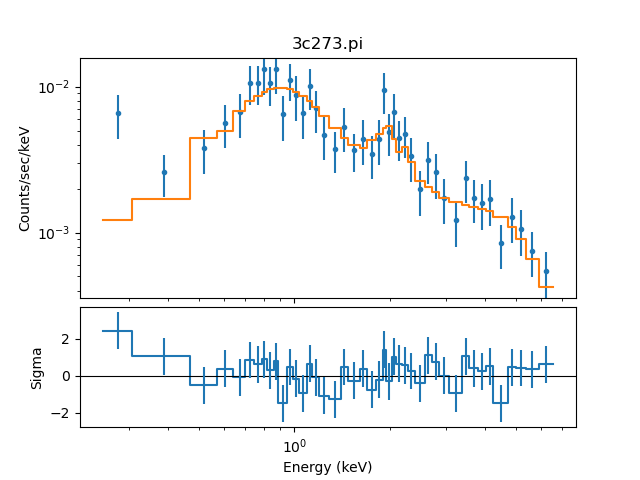### Figure 3: Fit and sigma residuals

In CIAO 4.13 the display of model fits to PHA data—that is, in the plots plot_fit, plot_model, plot_model_component, plot_source, and plot_source_component—have changed to use a "histogram" style rather than lines connecting the center of each bin. The Y axis of residual-style plots have also been adjusted to always use a linear scale, no-matter the ylog setting for the plot.

The plot can be modified using matplotlib pyplot functions directly.

## Examining Fit Results

### Goodness of fit

The show_fit and get_fit_results commands allow access to the best-fit values and detailed information after the fit has been performed:

sherpa> show_fit()
Optimization Method: LevMar
name     = levmar
ftol     = 1.1920928955078125e-07
xtol     = 1.1920928955078125e-07
gtol     = 1.1920928955078125e-07
maxfev   = None
epsfcn   = 1.1920928955078125e-07
factor   = 100.0
numcores = 1
verbose  = 0

Statistic: Chi2Gehrels
Chi Squared with Gehrels variance.

The variance is estimated from the number of counts in each bin,
but unlike Chi2DataVar, the Gaussian approximation is not
used. This makes it more-suitable for use with low-count data.

The standard deviation for each bin is calculated using the
approximation from _:

sigma(i,S) = 1 + sqrt(N(i,s) + 0.75)

where the higher-order terms have been dropped. This is accurate
to approximately one percent. For data where the background has
not been subtracted then the error term is:

sigma(i) = sigma(i,S)

whereas with background subtraction,

sigma(i)^2 = sigma(i,S)^2 + [A(S)/A(B)]^2 sigma(i,B)^2

A(B) is the off-source "area", which could be
the size of the region from which the background is extracted, or
the length of a background time segment, or a product of the two,
etc.; and A(S) is the on-source "area". These terms may be defined
for a particular type of data: for example, PHA data sets A(B) to
BACKSCAL * EXPOSURE from the background data set and A(S) to
BACKSCAL * EXPOSURE from the source data set.

--------
Chi2DataVar, Chi2ModVar, Chi2XspecVar

Notes
-----
The accuracy of the error term when the background has been
subtracted has not been determined. A preferable approach to
background subtraction is to model the background as well as the
source signal.

References
----------

..  "Confidence limits for small numbers of events in
astrophysical data", Gehrels, N. 1986, ApJ, vol 303,
p. 336-346.

Fit:Dataset               = 1
Method                = levmar
Statistic             = chi2gehrels
Initial fit statistic = 710.713
Final fit statistic   = 29.3673 at function evaluation 19
Data points           = 43
Degrees of freedom    = 41
Probability [Q-value] = 0.9124
Reduced statistic     = 0.716276
Change in statistic   = 681.345
p1.gamma       2.13925      +/- 0.078812
p1.ampl        0.000220978  +/- 1.44103e-05

# retrieve a single value with get_fit_results:
sherpa> fitres = get_fit_results()
sherpa> print(fitres.qval)
0.9123996703894587
sherpa> print(fitres.rstat)
0.7162758676095534


The number of bins in the fit (Data points), the number of degrees of freedom, i.e. the number of bins minus the number of free parameters, and the final fit statistic value are reported. If the chosen statistic is one of the χ2 statistics, as in this example, the reduced statistic (i.e. the statistic value divided by the number of degrees of freedom) and the probability (Q-value) are included as well.

The calc_chisqr command calculates the statistic contribution per bin:

sherpa> calc_chisqr()
array([5.92219689e+00, 1.15095438e+00, 2.67144137e-01, 1.51313185e-01,
5.57811410e-03, 7.39920674e-01, 3.84220524e-01, 7.93894437e-01,
8.92233791e-02, 6.43705828e-01, 2.20535462e+00, 2.31863201e-01,
2.37124647e-02, 9.02061151e-01, 4.35570832e-01, 6.67330704e-03,
1.17580452e+00, 1.61370022e+00, 2.31836814e-01, 6.96734907e-02,
1.55987296e-01, 5.55911748e-01, 4.24866413e-02, 1.99746379e+00,
9.33450840e-02, 1.06955971e+00, 4.45717186e-01, 3.32144464e-01,
6.90133232e-02, 1.52576625e-01, 1.24063898e+00, 5.91068198e-01,
1.31817201e-04, 8.78320376e-01, 1.11984115e+00, 1.59891448e-01,
5.79323503e-02, 2.60885837e-01, 2.20699877e+00, 2.04946799e-01,
1.82494758e-01, 1.20470103e-01, 3.85081946e-01])


### Confidence intervals

The covariance() command—which may be shortened to covar()—computes covariance matrices and provides an estimate of confidence intervals for the thawed parameters; also see the related command conf():

sherpa> covar()
Dataset               = 1
Confidence Method     = covariance
Iterative Fit Method  = None
Fitting Method        = levmar
Statistic             = chi2gehrels
covariance 1-sigma (68.2689%) bounds:
Param            Best-Fit  Lower Bound  Upper Bound
-----            --------  -----------  -----------
p1.gamma          2.13925   -0.0815616    0.0815616
p1.ampl       0.000220978 -1.46038e-05  1.46038e-05

sherpa> conf()
p1.gamma lower bound:   -0.0815616
p1.ampl lower bound:    -1.46038e-05
p1.ampl upper bound:    1.46038e-05
p1.gamma upper bound:   0.0821867
Dataset               = 1
Confidence Method     = confidence
Iterative Fit Method  = None
Fitting Method        = levmar
Statistic             = chi2gehrels
confidence 1-sigma (68.2689%) bounds:
Param            Best-Fit  Lower Bound  Upper Bound
-----            --------  -----------  -----------
p1.gamma          2.13925   -0.0815616    0.0821867
p1.ampl       0.000220978 -1.46038e-05  1.46038e-05


The output is the best-fit parameter value with positive and negative error estimates.

### Sensitivity to a single parameter

The int_proj function can be used to how the search statistic varies with a parameter, which lets us see how much we can trust the error analysis (a nice smooth curve centered at the best-fit location is good, and one with a lot of structure is not).

sherpa> int_proj(p1.gamma)


### Figure 4: How does the statistic vary with the gamma parameter?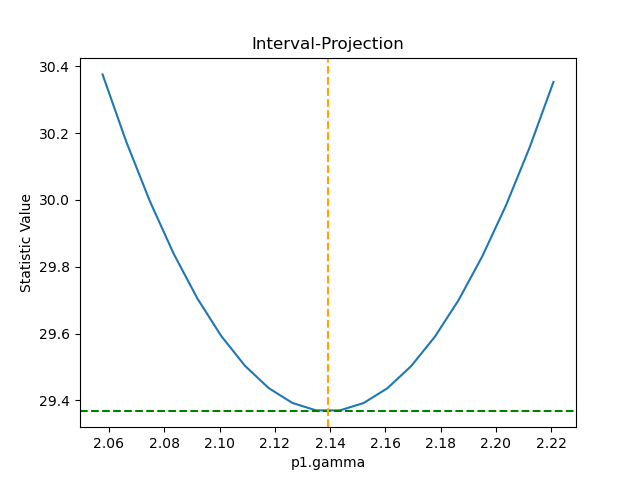### Figure 4: How does the statistic vary with the gamma parameter?

The vertical orange dashed line indicates the best-fit location for the parameter and the horizontal green dashed line the statistic for the best-fit location. In this example we can see that the statistic increases to both sides, and is approximately symmetric.

The range chosen here corresponds to a change in statistic of $$\sim 1$$, which corresponds to $$1 \sigma$$. The range over which the calculation can be changed to explore a larger range, such as with:

sherpa> int_proj(p1.gamma, min=2, max=2.3, nloop=51)


### Sensitivity to two parameters

The reg_proj function lets us see how two parameters are related:

sherpa> reg_proj(p1.gamma, p1.ampl)


### Figure 5: How does the statistic vary with the gamma and amplitude parameters?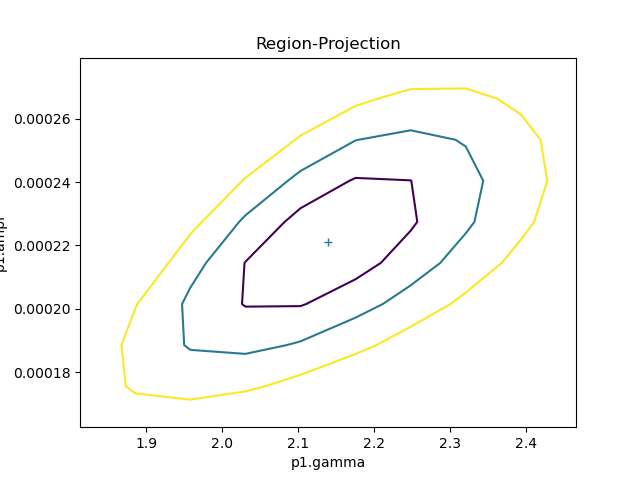### Figure 5: How does the statistic vary with the gamma and amplitude parameters?

As there are now two parameters being varied, unlike the previous section, the statistic value is displayed as a contour plot. There are three contours, correspnding to $$1 \sigma$$ (smallest, purple), $$2 \sigma$$ (blue-ish), and $$3 \sigma$$ (largest, yellow) away from the best-fit location (indicated by the cross).

As with the one-dimensional analysis (int_proj) we can explicitly give the range and binning to use. Here we increase the number of bins (the default is 10 along each axis) to smooth out the contours:

sherpa> reg_proj(p1.gamma, p1.ampl, min=[1.8, 1.6e-4], max=[2.5,2.8e-4], nloop=[41, 41])


### Figure 6: Tweaking the range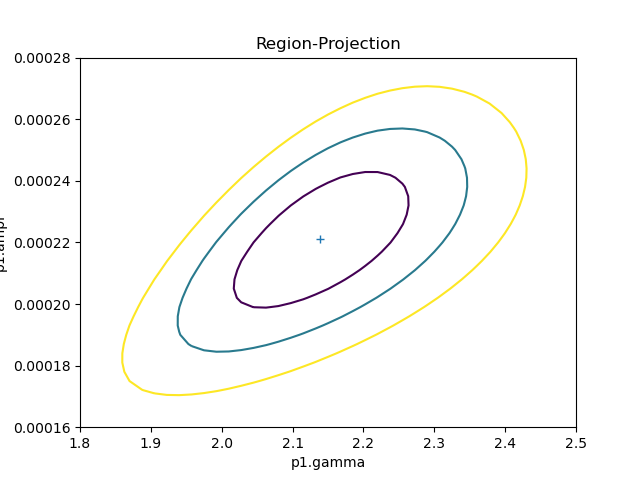### Figure 6: Tweaking the range

In this case we have explicitly chosen the range for each axis and the number of bins (that is, the step size along the axis). As we use more points than the previous version (Figure 5) but keep the range similar we can better trace the contour shapes.

For this example the analysis is fast, as there are no extra parameters to fit at each iteration. As soon as there are the run-time of reg+proj can increase significantly!

### Flux and CountsTip

Please review the How can we calculate a flux in Sherpa? analysis guide on the CIAO site.

To calculate the flux of a PHA data set, use the calc_photon_flux and calc_energy_flux commands. The flux may be calculated over the entire data set or over a specific range:

sherpa> calc_photon_flux()
0.00047016461535720494

sherpa> calc_photon_flux(2., 10.)
7.317496311869523e-05

sherpa> calc_energy_flux()
9.651851646748475e-13

sherpa> calc_energy_flux(2., 10.)
4.599863613722727e-13


To calculate the counts of a PHA data set, use the calc_data_sum, calc_model_sum, or calc_source_sum commands. As with the flux calculations, these commands may be given a range:

sherpa> calc_data_sum()
706.8571409201713

sherpa> calc_data_sum(2., 10.)
306.2301570173737

sherpa> calc_model_sum()
637.794750679389

sherpa> calc_model_sum(2., 10.)
272.49939336502973

sherpa> calc_source_sum()
0.047016461847183715

sherpa> calc_source_sum(2., 10.)
0.007317496309896684Warning

These functions accept any range of values but they can only be relied on for values that lie wthin the instrument energy range (so roughly 0.1 to 12 keV for Chandra ACIS data using a default setup).

## Scripting It

The file fit.py is a Python script which performs the primary commands used above; it can be executed by typing %run -i fit.py on the Sherpa command line.

The Sherpa script command may be used to save everything typed on the command line in a Sherpa session:

sherpa> script(filename="sherpa.log", clobber=False)


(Note that restoring a Sherpa session from such a file could be problematic since it may include syntax errors, unwanted fitting trials, et cetera.)

## History

 14 Nov 2007 rewritten for CIAO 4.0 Beta 3 29 Apr 2008 show_all command is available in CIAO 4.0 09 Dec 2008 figures moved inline with text 09 Dec 2008 updated for Sherpa 4.1 16 Feb 2009 example of guess functionality added 29 Apr 2009 new script command is available with CIAO 4.1.2 15 Dec 2009 updated for CIAO 4.2 09 Jul 2010 updated for CIAO 4.2 Sherpa v2: S-Lang version of thread removed 15 Dec 2010 updated for Sherpa in CIAO 4.3: use of log_scale replaced with set_xlog/set_ylog; list_models is available with new argument options; new functions calc_stat_info and get_stat_info return goodness-of-fit information 15 Dec 2011 reviewed for CIAO 4.4 (no changes) 13 Dec 2012 updated for CIAO 4.5: background data may now be filtered separately from associated source data using the new bkg_id argument of the notice_id/ignore_id commands 04 Jun 2013 added a paragraph on statistical and systematic errors to the section "Load the Spectrum and Instrument Responses". Made small edits to the text. 03 Dec 2013 reviewed for CIAO 4.6 06 Apr 2015 updated for CIAO 4.7, no content change 01 Dec 2015 updated for CIAO 4.8, outputs updated 01 Dec 2015 updated for CIAO 4.9, updated for Python 3 compatibility. 11 Apr 2018 updated for CIAO 4.10, outputs updated 04 Dec 2018 updated for CIAO 4.11, outputs updated 09 Dec 2019 updated for CIAO 4.12, ChIPS figures replaced with matplotlib 15 Dec 2020 updated for CIAO 4.13: plot style has been updated, the default energy range changed to 0.3-6 keV, and two new sections have been added: Sensitivity to a single parameter and Sensitivity to two parameters. 01 Mar 2022 updated for CIAO 4.14, added note about the "+/-" uncertainties for fits using the Levenberg-Marquardt optimizer. 28 Nov 2022 updated screen output for CIAO 4.15.